#### Multiple Loops in Excel Vba

In this article we are going to learn how to use multiple loops in VBA. Two commonly used loops in VBA are the For loop and the For Each loop. We are going to use the For Next Loop first.

To add a command button click on “Insert” and then select “Command Button” from ActiveX group.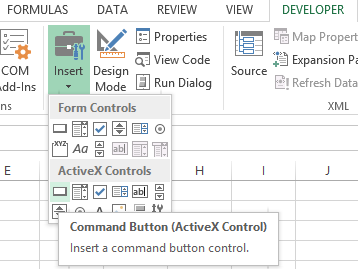Create a command button.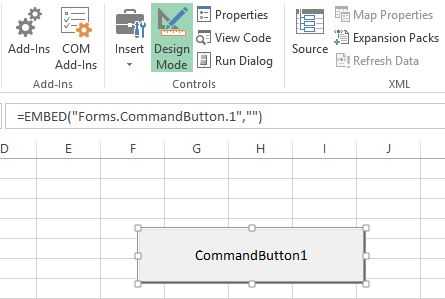Write click on the command button and Select “View Code”.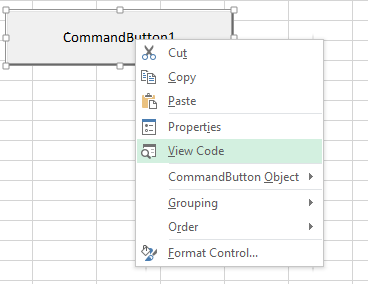## Nesting Vba Loops

Write this line of code. After writing the code, close the window by clicking on the red X button on the top left of the screen.

```Dim i As Integer, j As Integer
For i=1 To 1 to 6
For j=1 To 2
Cells (i, j).Value=100
Next j
Next i
End Sub```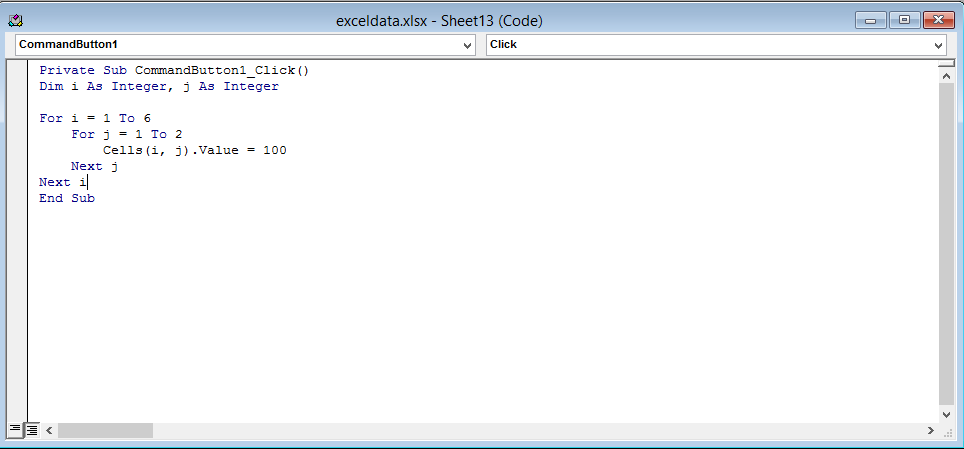Click on the command button to see this result.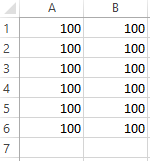This is how to implement two for loops using Excel VBA.

## Nesting both For and For Each Loops

Here’s an example of using both loops in a single macro:

`Sub MultipleLoops()`

` `

`Dim i As Integer `

`Dim j As Integer `

`Dim cell As Range `

`For i = 1 To 5 'First loop to iterate through 5 rows `

`    For j = 1 To 3 'Second loop to iterate through 3 columns `

`        'Get the current cell in the current row and column `

`        Set cell = Cells(i, j) `

`        'Add the values of the current cell and the next cell in the same row `

`        cell.Value = cell.Value + Cells(i, j + 1).Value `

`    Next j `

`Next i `

`End Sub`

In this example, the first loop (For i = 1 to 5) iterates through five rows, while the second loop (For j = 1 to 3) iterates through three columns in each row. The inner loop (For j = 1 to 3) performs an operation on each cell in the current row, and the outer loop (For i = 1 to 5) moves to the next row after the inner loop has completed. This allows the macro to perform the operation on all 15 cells in the range.